# Solution May 25, 2007

### Problem

Given natural numbers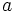$a\,$,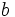$b\,$ and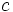$c\,$ that are pairwise distinct and satisfy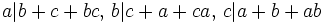$a | b+c+bc,\, b | c+a+ca,\, c | a+b+ab\,$,

prove that at least one of the numbers$a\,$,$b\,$,$c\,$ is not prime.

### Solution

Assume that a,b,c are distinct primes. Further assume without loss of generality that a < b < c.

Note that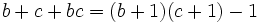$b+c+bc = (b+1)(c+1)-1\,$, so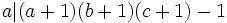$a|(a+1)(b+1)(c+1)-1\,$ and similar for$b\,$ and$c\,$. Also,$a,\, b,\, c$ are pairwise coprime, so we have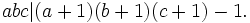$abc | (a+1)(b+1)(c+1)-1.\,$

Let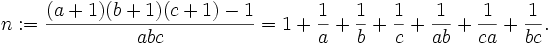$n := \frac{(a+1)(b+1)(c+1)-1}{abc} = 1 + \frac 1a + \frac 1b + \frac 1c + \frac 1{ab} + \frac 1{ca} + \frac 1{bc}.\,$.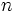$n\,$ is an integer. Obviously,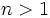$n>1\,$. The right side is a strictly decreasing function in all three variables. Let's check a few cases:

• if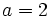$a = 2\,$ and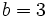$b = 3\,$, the right side becomes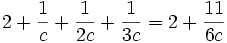$2 + \frac1{c} + \frac1{2c} + \frac1{3c} = 2 + \frac{11}{6c}\,$ which is never an integer for any$c\,$
• if$a = 2\,$,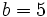$b = 5\,$, the right side is not an integer for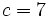$c=7\,$ and less than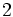$2\,$ for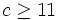$c\geq 11\,$, so it's not an integer then either.
• if$a = 2\,$,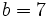$b=7\,$ then$c\geq 11\,$ and again,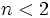$n<2\,$.
• if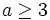$a \geq 3\,$, then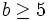$b\geq 5\,$ and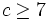$c\geq 7\,$ and, (surprise!)$n<2\,$.

This shows that$n\,$ can never be an integer, in contradiction with our assumptions, completing the proof.